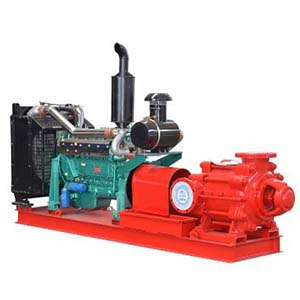The Basic Parameters that Characterize the Pump Performance

2018-12-17

The basic parameters that characterize the main performance of the pump are as follows:

1, flow Q
Flow is the amount (volume or mass) of liquid that the pump delivers per unit of time.
The volume flow rate is expressed by Q, and the unit is: m3/s, m3/h, l/s, and the like.
The mass flow rate is expressed by Qm, and the unit is: t/h, kg/s, and the like.
The relationship between mass flow and volume flow is:
Qm=ρQ
Where ρ is the density of the liquid (kg/m3, t/m3), and the normal temperature is ρ=1000kg/m3.
The lift is the increase in energy per unit weight of liquid pumped from the pump inlet (pump inlet flange) to the pump outlet (pump outlet flange). That is, the effective energy obtained by a Newtonian liquid through a pump. The unit is N·m/N=m, which is the height of the liquid column of the pump pumping liquid.
3, speed n
The rotational speed is the number of revolutions per unit time of the pump shaft, denoted by the symbol n, and the unit is r/min.
4, NPSH NPSH
The NPSH margin is also called the net positive nozzle, which is the main parameter indicating the cavitation performance. The NPSH has been expressed in China by Δh.5, power and efficiency
The power of the pump usually refers to the input power, that is, the power on the shaft of the prime mover pump, so it is also called the shaft power, which is denoted by P;
The effective power of the pump, also known as the output power, is represented by Pe. It is the effective energy that the liquid delivered from the pump per unit time gets in the pump.
Because the head is the effective energy obtained by pumping the unit weight of liquid from the pump, the product of the head and the mass flow and the gravitational acceleration is the effective energy obtained from the liquid output from the pump per unit time—the pump Effective power:
Pe=ρgQH(W)=γQH(W)
Where ρ is the density of the liquid delivered by the pump (kg/m3);
Γ——the severity of the liquid delivered by the pump (N/m3);
Q——the flow rate of the pump (m3/s);
H——the head of the pump (m);
G——gravitational acceleration (m/s2).
The difference between the shaft power P and the effective power Pe is the loss power in the pump, and its magnitude is measured by the efficiency of the pump. The efficiency of the pump is the ratio of effective power to shaft power, expressed as η.WhatsApp me